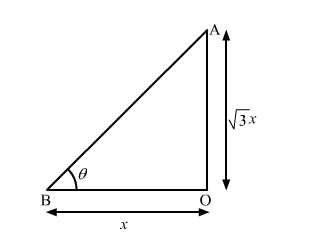# Choose the correct answer of the following question:`
Question:

Choose the correct answer of the following question:

If the height of a vertical pole is $\sqrt{3}$ times the length of its shadow on the ground, then the angle of elevation of the sun at that time is

(a) 30°                    (b) 45°                     (c) 60°                     (d) 75°

Solution:Here, $A O$ be the pole; $B O$ be its shadow and $\theta$ be the angle of elevation of the sun.

Let $\mathrm{BO}=x$

Then, $\mathrm{AO}=x \sqrt{3}$

In $\Delta \mathrm{AOB}$,

$\tan \theta=\frac{\mathrm{AO}}{\mathrm{BO}}$

$\Rightarrow \tan \theta=\frac{x \sqrt{3}}{x}$

$\Rightarrow \tan \theta=\sqrt{3}$

$\Rightarrow \tan \theta=\tan 60^{\circ}$

$\therefore \theta=60^{\circ}$

Hence, the correct answer is option (c).